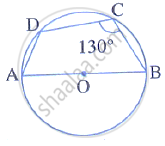Share

# In the Given Figure, Ab is the Diameter of a Circle with Centre O. ∠Bcd = 130°. Find: (I) ∠Dab (Ii) ∠Dba - Mathematics

Course

#### Question

In the given figure, AB is the diameter of a circle with centre O. ∠BCD = 130°. Find:
(i) ∠DAB
(ii) ∠DBA#### Solution

i. ABCD is a cyclic quadrilateral
m∠DAB = 180° − ∠DCB
= 180° - 130°
= 50°

m∠DAB + m∠ADB + m∠DBA = 180°
⇒ 50° + 90° + m∠DBA = 180°
⇒ m∠DBA = 40°

Is there an error in this question or solution?